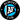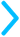Going back and forth between fractions, decimals, and percents can get confusing, and you can use our fraction-decimal convertor or decimal-percent convertor to convert anything you need.

But, there are about 15 conversions you should just memorize. Use the flashcards and chart below to study!## Quiz Yourself

Click the card to reveal the equivalent decimal, percent, or fraction.

$\frac{1}{10}$
$0.1$$10\%$

## Fraction to Decimal to Percent Chart

FractionDecimalPercent
$\frac{1}{10}$$0.1$$10\%$
$\frac{1}{5}$$0.2$$20\%$
$\frac{1}{4}$$0.25$$25\%$
$\frac{3}{10}$$0.3$$30\%$
$\frac{1}{3}$$0.33$$33\%$
$\frac{2}{5}$$0.4$$40\%$
$\frac{1}{2}$$0.50$$50\%$
$\frac{3}{5}$$0.6$$60\%$
$\frac{2}{3}$$0.67$$67\%$
$\frac{7}{10}$$0.7$$70\%$
$\frac{3}{4}$$0.75$$75\%$
$\frac{4}{5}$$0.8$$80\%$
$\frac{9}{10}$$0.9$$90\%$